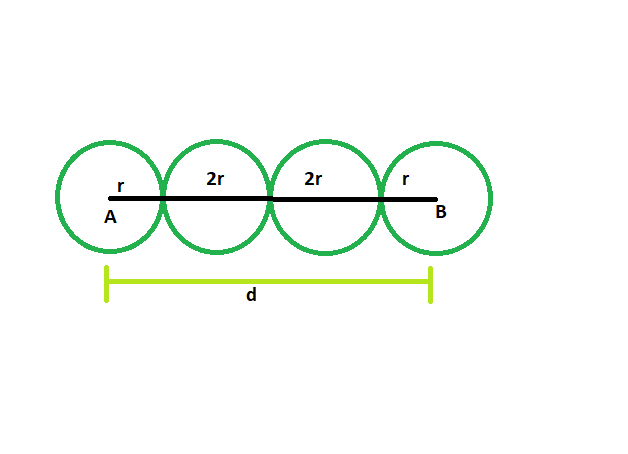GeeksforGeeks App
Open AppBrowser
Continue

# Find the radius of the circles which are lined in a row, and distance between the centers of first and last circle is given

Given here are n circles which touch each other externally, and are lined up in a row. The distance between the centers of the first and last circle is given. The circles have a radius of equal length. The task is to find the radius of each circle.
Examples:

```Input: d = 42, n = 4
Output: The radius of each circle is 7

Input: d = 64, n = 5
Output: The radius of each circle is 8```Approach
Suppose there are n circles each having radius of length r
Let, the distance between the first and last circles = d
From the figure, it is clear,
r + r + (n-2)*2r = d
2r + 2nr – 4r = d
2nr – 2r = d
so, r = d/(2n-2)## C++

 `// C++ program to find radii of the circles``// which are lined in a row``// and distance between the``// centers of first and last circle is given` `#include ``using` `namespace` `std;` `void` `radius(``int` `n, ``int` `d)``{``    ``cout << ``"The radius of each circle is "``         ``<< d / (2 * n - 2) << endl;``}` `// Driver code``int` `main()``{``    ``int` `d = 42, n = 4;``    ``radius(n, d);``    ``return` `0;``}`

## Java

 `// Java program to find radii of the circles``// which are lined in a row``// and distance between the``// centers of first and last circle is given``import` `java.io.*;` `class` `GFG``{` `static` `void` `radius(``int` `n, ``int` `d)``{``    ``System.out.print( ``"The radius of each circle is "``        ``+d / (``2` `* n - ``2``));``}` `// Driver code``static` `public` `void` `main (String []args)``{``    ``int` `d = ``42``, n = ``4``;``    ``radius(n, d);``}``}` `// This code is contributed by anuj_67..`

## Python3

 `# Python program to find radii of the circles``# which are lined in a row``# and distance between the``# centers of first and last circle is given` `def` `radius(n, d):``    ``print``(``"The radius of each circle is "``,``        ``d ``/` `(``2` `*` `n ``-` `2``));` `d ``=` `42``; n ``=` `4``;``radius(n, d);`  `# This code is contributed by PrinciRaj1992`

## C#

 `// C# program to find radii of the circles``// which are lined in a row``// and distance between the``// centers of first and last circle is given``using` `System;` `class` `GFG``{` `static` `void` `radius(``int` `n, ``int` `d)``{``    ``Console.Write( ``"The radius of each circle is "``        ``+d / (2 * n - 2));``}` `// Driver code``static` `public` `void` `Main ()``{``    ``int` `d = 42, n = 4;``    ``radius(n, d);``}``}` `// This code is contributed by anuj_67..`

## Javascript

 ``

Output:

`The radius of each circle is 7`

Time Complexity: O(1)

Auxiliary Space: O(1)

My Personal Notes arrow_drop_up